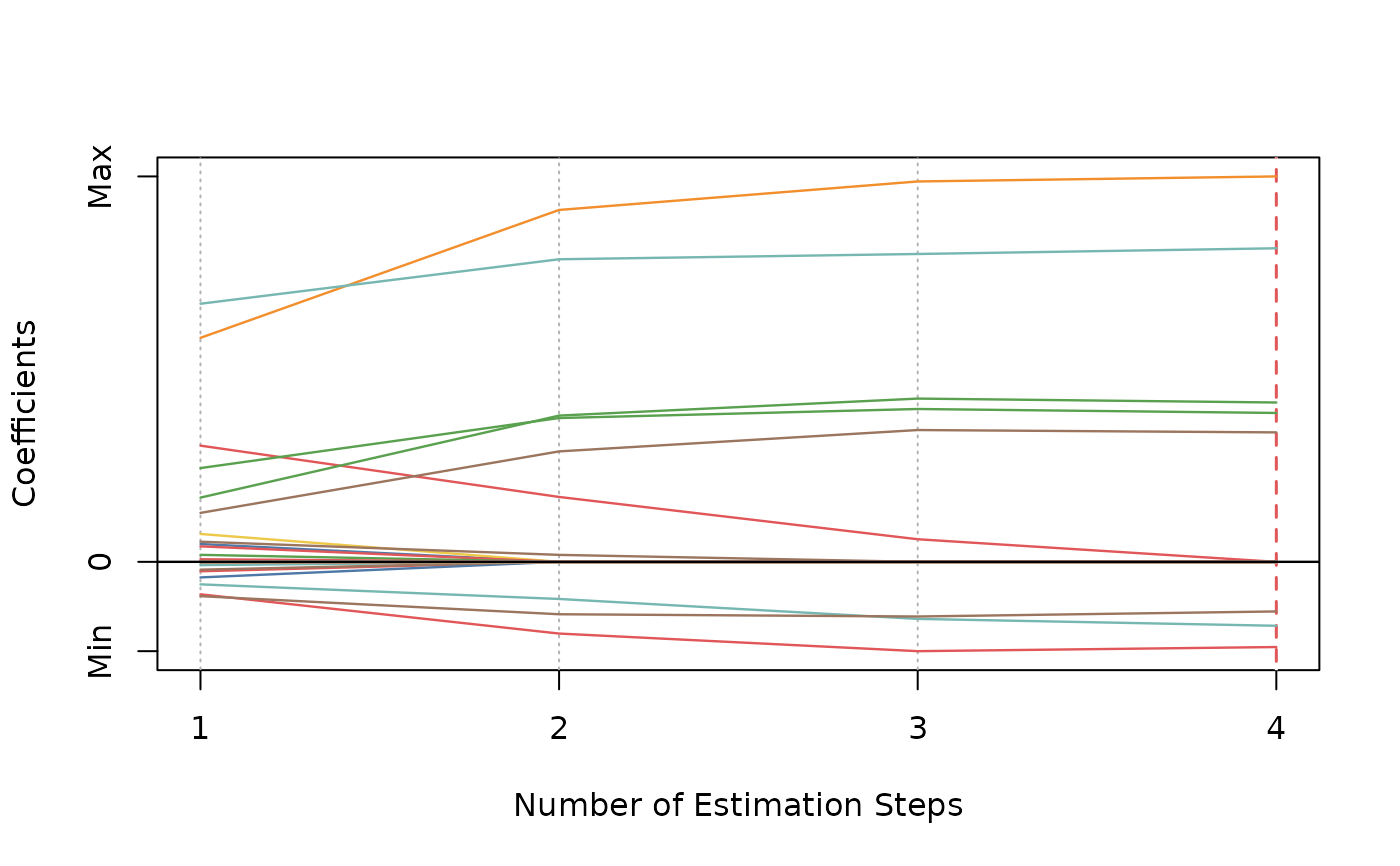Multi-Step Adaptive Elastic-Net

msaenet(x, y, family = c("gaussian", "binomial", "poisson", "cox"),
init = c("enet", "ridge"), alphas = seq(0.05, 0.95, 0.05),
tune = c("cv", "ebic", "bic", "aic"), nfolds = 5L,
rule = c("lambda.min", "lambda.1se"), ebic.gamma = 1, nsteps = 2L,
tune.nsteps = c("max", "ebic", "bic", "aic"), ebic.gamma.nsteps = 1,
scale = 1, lower.limits = -Inf, upper.limits = Inf,
penalty.factor.init = rep(1, ncol(x)), seed = 1001,
parallel = FALSE, verbose = FALSE)

## Arguments

x Data matrix. Response vector if family is "gaussian", "binomial", or "poisson". If family is "cox", a response matrix created by Surv. Model family, can be "gaussian", "binomial", "poisson", or "cox". Type of the penalty used in the initial estimation step. Can be "enet" or "ridge". See glmnet for details. Vector of candidate alphas to use in cv.glmnet. Parameter tuning method for each estimation step. Possible options are "cv", "ebic", "bic", and "aic". Default is "cv". Fold numbers of cross-validation when tune = "cv". Lambda selection criterion when tune = "cv", can be "lambda.min" or "lambda.1se". See cv.glmnet for details. Parameter for Extended BIC penalizing size of the model space when tune = "ebic", default is 1. For details, see Chen and Chen (2008). Maximum number of adaptive estimation steps. At least 2, assuming adaptive elastic-net has only one adaptive estimation step. Optimal step number selection method (aggregate the optimal model from the each step and compare). Options include "max" (select the final-step model directly), or compare these models using "ebic", "bic", or "aic". Default is "max". Parameter for Extended BIC penalizing size of the model space when tune.nsteps = "ebic", default is 1. Scaling factor for adaptive weights: weights = coefficients^(-scale). Lower limits for coefficients. Default is -Inf. For details, see glmnet. Upper limits for coefficients. Default is Inf. For details, see glmnet. The multiplicative factor for the penalty applied to each coefficient in the initial estimation step. This is useful for incorporating prior information about variable weights, for example, emphasizing specific clinical variables. To make certain variables more likely to be selected, assign a smaller value. Default is rep(1, ncol(x)). Random seed for cross-validation fold division. Logical. Enable parallel parameter tuning or not, default is FALSE. To enable parallel tuning, load the doParallel package and run registerDoParallel() with the number of CPU cores before calling this function. Should we print out the estimation progress?

## Value

List of model coefficients, glmnet model object, and the optimal parameter set.

Nan Xiao and Qing-Song Xu. (2015). Multi-step adaptive elastic-net: reducing false positives in high-dimensional variable selection. Journal of Statistical Computation and Simulation 85(18), 3755--3765.

## Examples

dat <- msaenet.sim.gaussian(
n = 150, p = 500, rho = 0.6,
coef = rep(1, 5), snr = 2, p.train = 0.7,
seed = 1001
)

msaenet.fit <- msaenet(
dat$x.tr, dat$y.tr,
alphas = seq(0.2, 0.8, 0.2),
nsteps = 3L, seed = 1003
)

print(msaenet.fit)#> Call: msaenet(x = dat$x.tr, y = dat$y.tr, alphas = seq(0.2, 0.8, 0.2),
#>     nsteps = 3L, seed = 1003)
#>   Df      %Dev      Lambda
#> 1  8 0.7941158 1.34921e+15msaenet.nzv(msaenet.fit)#>    2   4   5  35 114 269 363 379msaenet.fp(msaenet.fit, 1:5)#>  5msaenet.tp(msaenet.fit, 1:5)#>  3msaenet.pred <- predict(msaenet.fit, dat$x.te) msaenet.rmse(dat$y.te, msaenet.pred)#>  2.821818plot(msaenet.fit)